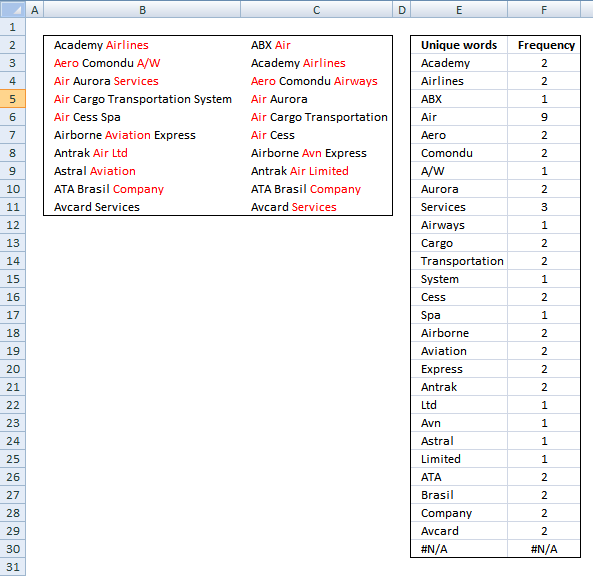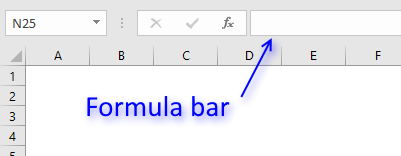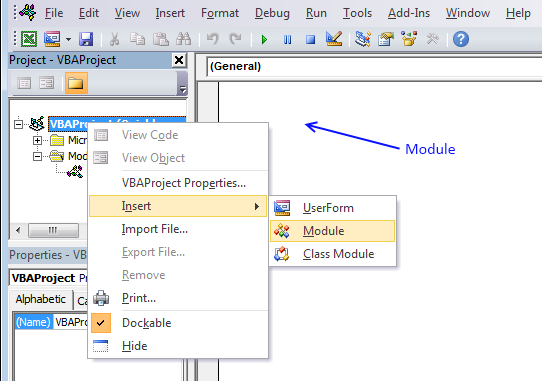Author: Oscar Cronquist Article last updated on January 15, 2019This user defined function creates a unique distinct list of words and how many times they occur in the selected range.

User defined Function Syntax

FREQWORDS(cell_range, position)

Arguments

 cell_range Required. The range you want to use. position Required. Which column to return. The first column contains the values and the second column contains their corresponding frequency.

Example

Array formula in cell E3:E30:

=FreqWords(B2:C11, 1)

Array formula in cell F3:F30:

=FreqWords(B2:C11, 2)

How to create an array formula

1. Select cell range E3:E30.
2. Copy (Ctrl + c) array formula and paste (Ctrl + v)  to formula bar.3. Press and hold Ctrl + Shift simultaneously.
4. Press Enter once.
5. Release all keys.

VBA

'Name function and declare arguments
Function FreqWords(tbl_array As Range, pos As Integer) As Variant()
'Declare variables and their data types
Dim cell As Variant, wrds As Variant, i As Integer
Dim a As Integer, j As Integer
Dim tmp() As String, nr() As Integer
'Redimension variable tmp so it can grow using Redim Preserve
ReDim tmp(0), nr(0)

'Assign 1 to first value in array variable nr
nr(0) = 1
'Iterate through cells in cell range
For Each cell In tbl_array
'Split words in cell
wrds = Split(cell)
'Iterate thorugh words
For i = 0 To UBound(wrds)
'Iterate through arrayvariable tmp
For j = 0 To UBound(tmp)
'If variable wrds equal variable tmp then increase value in variable nr by 1
If wrds(i) = tmp(j) Then
nr(j) = nr(j) + 1
a = 1
Exit For
End If
Next j
'Check if a is not equal to 1
If a <> 1 Then
'Copy value from variable wrds to tmp
tmp(UBound(tmp)) = wrds(i)
'Add another container to array variable tmp
ReDim Preserve tmp(UBound(tmp) + 1)
ReDim Preserve nr(UBound(tmp))
nr(UBound(tmp)) = 1
End If
a = 0
Next i
Next cell
'Return values in column 1 if argument pos is equal to 1
If pos = 1 Then
ReDim Preserve tmp(UBound(tmp) - 1)
FreqWords = Application.Transpose(tmp)
Else
'Return values in column 2 if argument pos is not equal to 1
ReDim Preserve nr(UBound(nr) - 1)
FreqWords = Application.Transpose(nr)
End If
End Function

How to add the User defined Function to your workbook1. Press Alt-F11 to open visual basic editor
2. Click Module on the Insert menu
3. Copy and paste vba code
4. Exit visual basic editor